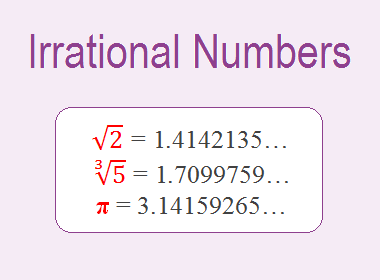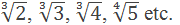# Irrational Numbers## Define Irrational Numbers with Examples.

Definition: A decimal number which is non-terminating and non-recurring is called an irrational number. Irrational numbers cannot be expressed in the form of p/q where p and q are integers and q≠0. The square root of all non-square numbers, cube root of all non-cube numbers, etc. are all non-terminating and non-recurring decimal numbers, so they are irrational numbers. π(pi) also is an irrational number.

Here are some examples:

√2 = 1.4142135…

√3 = 1.7320508…

√5 = 2.2360679…

√7 = 2.6457513…

π = 3.14159265…

are non-terminating and non-recurring decimal numbers, so they are irrational numbers.

Some other examples of irrational numbers are:********************

10 Math Problems officially announces the release of Quick Math Solver, an Android App on the Google Play Store for students around the world.

********************

### Properties of irrational numbers:

Here are some of the properties of irrational numbers:

1. Irrational numbers are non-terminating and non-recurring decimal numbers.

2. Irrational numbers are closed under the operation of addition i.e. the sum of two irrational numbers is always an irrational number.

3. Irrational numbers are closed under the operation of subtraction i.e. the difference of two irrational numbers is always an irrational number.

4. Irrational numbers are not closed under the operation of multiplication i.e. the product of two irrational numbers may not be an irrational number.

5. Irrational numbers are not closed under the operation of division. i.e. the quotient of two irrational numbers may not be an irrational number.

### What are the differences between rational and irrational numbers?

Following are the differences between rational and irrational numbers:

1. Rational numbers can be expressed in the form of p/q where q ≠ 0 and p and q are integers, whereas irrational numbers cannot be expressed in such form.

2. Rational numbers may be terminating or non-terminating but recurring decimal numbers whereas irrational numbers are always non-terminating and non-recurring decimal numbers.

3. Rational numbers are closed under the operation of multiplication and division, but irrational numbers are not closed under the operation of multiplication and division.

#### Some Question and Answers on Irrational Numbers

Question: How is π(pi) an irrational number? Because it can be expressed in the form of p/q which is 22/7.

Answer: 22/7 is not the exact value of π(pi). This is an approximate value only. The exact value of π(pi) is 3.141592653589793238... which is non-terminating and non-recurring decimals. So, the exact value of π(pi) is an irrational number.

Question: Is the sum of two irrational numbers always irrational?

Answer: Yes, the sum of two irrational numbers is always an irrational number.

Question: Are all square roots irrational numbers?

Answer: Square roots of non-square numbers are all irrational numbers.

Question: Are irrational numbers closed under multiplication?

Answer: No, irrational numbers are not closed under multiplication.

Question: Are every irrational number is a real number?

Answer: Yes, every irrational number is a real number.

Question: What about the between rational and irrational numbers?

Answer: The difference between rational and irrational numbers is irrational numbers.

Question: What about the sum of rational and irrational numbers?

Answer: The sum of a rational and irrational number is always an irrational number.

Question: How many irrational numbers are there between 1 and 6?

Answer: There are infinitely many irrational numbers between any two integers. So there are infinitely many rational numbers between 1 and 6.

Question: Find at least two irrational numbers between 2 and 3.

Answer: √5 and √6 are two irrational numbers between 2 and 3.

Question: Find an irrational number between 3 and 4.

Answer: √10 is an irrational number between 3 and 4.

Question: Find an irrational number between 1 and 2.

Answer: √3 is an irrational number between 1 and 2.

Question: Find two irrational numbers between 0.5 and 0.55.

Answer: √0.26 and √0.27 are two irrational numbers between 0.5 and 0.55.

Question: Find two irrational between 0.7 and 0.77.

Answer: √50 and √51 are two irrational numbers between 0.7 and 0.77.

Question: What about the sum of a rational number and an irrational number?

Answer: The sum of a rational number and an irrational number is an irrational number.

Question: What about the difference between rational and irrational numbers?

Answer: The difference between rational and irrational numbers is an irrational number.

Question: Is the product of two irrational numbers always irrational?

Answer: No, the product of two irrational numbers is not always irrational. For example: √2 × √8 = √16 = 4 which is a rational number.

If you have any questions or problems regarding the Irrational Numbers, you can ask here, in the comment section below.

1.